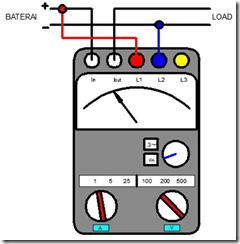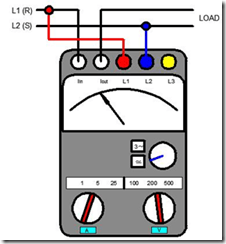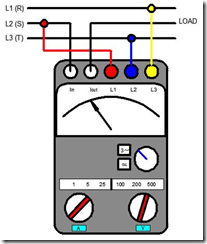Home > English > Watt Meter Function

# Watt Meter Function

a. Direct Current Power Measurement
Below is shown a picture of the wattmeter link for DC power measurement.Figure 48. The wattmeter linkage for DC power measurement

Wattmeter is an instrument of electrical power meter which is a combination of voltmeter and ampermeter. Direct current power measurements can be made using the Wattmeter gauge. In this instrument there are two kinds of coils that is the current coil and the voltage coil. The couples multiplied by the two coils are directly proportional to the multiplication of current and voltage.

b. Alternative Current Power Measurement
Below is shown a picture of a wattmeter link for one phase power measurement.Figure 49. Chart of the wattmeter relationship

for one phase power measurement Alternative current power measurements can be made using the Wattmeter gauge. In the picture above it can be seen that in connecting to the load and power supply line of wattmeter power for one phase power measurement there is similarity with DC power measurement of output input terminal at Wattmeter have in common with when measuring DC power.

At the measurement of electric power one-phase alternating current load is performed by using 4 terminal point I / O at Wattmeter ie terminal Iin, Iout, L1 and L2. Calculations need to be done as listed in the table provided above.

c. Alternative Current Power Measurement Phase Back Example:
In measuring the load of 1 phase switch current (I) at position number 5 next switchtegangan (V) at position 100 then C = 5 (according to table above), then if needle shows number 30 then power reading formulated as follows:
P = UIC
P = 30. 5. 5
P = 750 watts

Below is shown a picture of the wattmeter link for one phase power measurement.Figure 50. The wattmeter linkage for three phase power measurements"Tasikmalaya, Indonesia"